Springer Nature is making SARS-CoV-2 and COVID-19 research free. View research | View latest news | Sign up for updates

# Analysis of Pivot Sampling in Dual-Pivot Quicksort: A Holistic Analysis of Yaroslavskiy’s Partitioning Scheme

## Abstract

The new dual-pivot Quicksort by Vladimir Yaroslavskiy—used in Oracle’s Java runtime library since version 7—features intriguing asymmetries. They make a basic variant of this algorithm use less comparisons than classic single-pivot Quicksort. In this paper, we extend the analysis to the case where the two pivots are chosen as fixed order statistics of a random sample. Surprisingly, dual-pivot Quicksort then needs more comparisons than a corresponding version of classic Quicksort, so it is clear that counting comparisons is not sufficient to explain the running time advantages observed for Yaroslavskiy’s algorithm in practice. Consequently, we take a more holistic approach and give also the precise leading term of the average number of swaps, the number of executed Java Bytecode instructions and the number of scanned elements, a new simple cost measure that approximates I/O costs in the memory hierarchy. We determine optimal order statistics for each of the cost measures. It turns out that the asymmetries in Yaroslavskiy’s algorithm render pivots with a systematic skew more efficient than the symmetric choice. Moreover, we finally have a convincing explanation for the success of Yaroslavskiy’s algorithm in practice: compared with corresponding versions of classic single-pivot Quicksort, dual-pivot Quicksort needs significantly less I/Os, both with and without pivot sampling.

This is a preview of subscription content, log in to check access.

1. 1.

Note that the meaning of $$\mathcal {L}$$ is different in our previous work : therein $$\mathcal {L}$$ includes the last value index variable $$\ell$$ attains which is never used to access the array. The authors consider the new definition clearer and therefore decided to change it.

2. 2.

Total independence means that the joint probability function of all random variables factorizes into the product of the individual probability functions [4, p. 53], and does so not only pairwise.

3. 3.

We use the neologism “linearithmic” to say that a function has order of growth $$\Theta (n \log n)$$.

## References

1. 1.

Aumüller, M., Dietzfelbinger, M.: Optimal partitioning for dual pivot quicksort. In: Fomin, F.V., Freivalds, R., Kwiatkowska, M., Peleg, D. (ed.) International Colloquium on Automata, Languages and Programming, pp. 33–44. Springer, LNCS, vol 7965 (2013)

2. 2.

Bentley, J.L., McIlroy, M.D.: Engineering a sort function. Softw. Pract. Exp. 23(11), 1249–1265 (1993)

3. 3.

Chern, H.H., Hwang, H.K., Tsai, T.H.: An asymptotic theory for cauchy-euler differential equations with applications to the analysis of algorithms. J. Algorithms 44(1), 177–225 (2002)

4. 4.

Chung, K.L.: A Course in Probability Theory, 3rd edn. Academic Press, Waltham (2001)

5. 5.

Cormen, T.H., Leiserson, C.E., Rivest, R.L., Stein, C.: Introduction to Algorithms, 3rd edn. MIT Press, Cambridge (2009)

6. 6.

David, H.A., Nagaraja, H.N.: Order Statistics, 3rd edn. Wiley-Interscience, New York (2003)

7. 7.

Durand, M.: Asymptotic analysis of an optimized quicksort algorithm. Inform. Process. Lett. 85(2), 73–77 (2003)

8. 8.

Estivill-Castro, V., Wood, D.: A survey of adaptive sorting algorithms. ACM Comput. Surv. 24(4), 441–476 (1992)

9. 9.

Fill, J., Janson, S.: The number of bit comparisons used by quicksort: an average-case analysis. Elect. J. Prob. 17, 1–22 (2012)

10. 10.

Graham, R.L., Knuth, D.E., Patashnik, O.: Concrete Mathematics: A Foundation for Computer Science. Addison-Wesley, Boston (1994)

11. 11.

Hennequin, P.: Analyse en moyenne d’algorithmes : tri rapide et arbres de recherche. PhD Thesis, Ecole Politechnique, Palaiseau (1991)

12. 12.

Hennessy, J.L., Patterson, D.A.: Computer Architecture: A Quantitative Approach, 4th edn. Morgan Kaufmann Publishers, Burlington (2006)

13. 13.

Hoare, C.A.R.: Algorithm 65: Find. Commun. ACM 4(7), 321–322 (1961)

14. 14.

Kaligosi, K., Sanders, P.: How branch mispredictions affect quicksort. In: Erlebach, T., Azar, Y. (ed.) European Symposium on Algorithms, pp. 780–791. Springer, LNCS, vol 4168, (2006)

15. 15.

Kushagra, S., López-Ortiz, A., Qiao, A., Munro, J.I.: Multi-pivot quicksort: Theory and experiments. In: McGeoch, C.C., Meyer, U. (ed.) Meeting on Algorithm Engineering and Experiments, pp. 47–60. SIAM (2014)

16. 16.

LaMarca, A., Ladner, R.E.: The influence of caches on the performance of sorting. J. Algorithms 31(1), 66–104 (1999)

17. 17.

Mahmoud, H.M.: Sorting: A Distribution Theory. Wiley, New York (2000)

18. 18.

Martínez, C., Roura, S.: Optimal sampling strategies in quicksort and quickselect. SIAM J. Comput. 31(3), 683–705 (2001)

19. 19.

Martínez, C., Nebel, M.E., Wild, S.: Analysis of branch misses in quicksort. In: Sedgewick, R., Ward, M.D. (eds.) Meeting on Analytic Algorithmics and Combinatorics, pp. 114–128. SIAM, Philadelphia (2014)

20. 20.

Musser, D.R.: Introspective sorting and selection algorithms. Softw. Pract. Exp. 27(8), 983–993 (1997)

21. 21.

Nebel, M.E., Wild, S.: Pivot sampling in dual-pivot quicksort. In: Bousquet-Mélou, M., Soria, M. (ed.) International Conference on Probabilistic, Combinatorial and Asymptotic Methods for the Analysis of Algorithms, DMTCS-HAL Proceedings Series, vol BA, pp. 325–338 (2014)

22. 22.

Neininger, R.: On a multivariate contraction method for random recursive structures with applications to quicksort. Random Struct. Algorithms 19(3–4), 498–524 (2001)

23. 23.

Roura, S.: Improved master theorems for divide-and-conquer recurrences. J. ACM 48(2), 170–205 (2001)

24. 24.

Sedgewick, R.: Quicksort. PhD Thesis, Stanford University (1975)

25. 25.

Sedgewick, R.: The analysis of quicksort programs. Acta Inform. 7(4), 327–355 (1977)

26. 26.

Sedgewick, R.: Implementing quicksort programs. Commun. ACM 21(10), 847–857 (1978)

27. 27.

Vallée, B., Clément, J., Fill, J.A., Flajolet, P.: The number of symbol comparisons in quicksort and quickselect. In: Albers, S., Marchetti-Spaccamela, A., Matias, Y., Nikoletseas, S., Thomas, W. (ed.) International Colloquium on Automata, Languages and Programming, pp 750–763. Springer, LNCS, vol 5555, (2009)

28. 28.

van Emden, M.H.: Increasing the efficiency of quicksort. Commun. ACM 13(9), 563–567 (1970)

29. 29.

Wild, S.: Java 7’s Dual-Pivot Quicksort. Master thesis, University of Kaiserslautern (2012)

30. 30.

Wild, S., Nebel, M.E.: Average case analysis of Java 7’s dual pivot quicksort. In: Epstein, L., Ferragina, P. (ed.) European Symposium on Algorithms, pp. 825–836. Springer, LNCS, vol 7501, (2012)

31. 31.

Wild, S., Nebel, M.E., Reitzig, R., Laube, U.: Engineering Java 7’s dual pivot quicksort using MaLiJAn. In: Sanders, P., Zeh, N. (eds.) Meeting on Algorithm Engineering and Experiments, pp. 55–69. SIAM, Philadelphia (2013)

32. 32.

Wild, S., Nebel, M.E., Mahmoud, H.: Analysis of quickselect under Yaroslavskiy’s dual-pivoting algorithm. Algorithmica (to appear) (2014). doi:10.1007/s00453-014-9953-x

33. 33.

Wild, S., Nebel, M.E., Neininger, R.: Average case and distributional analysis of Java 7’s dual pivot quicksort. ACM Trans. Algorithms 11(3), 22:1–22:42 (2015)

## Author information

Correspondence to Sebastian Wild.

This work has been partially supported by funds from the Spanish Ministry for Economy and Competitiveness (MINECO) and the European Union (FEDER funds) under Grant COMMAS (ref. TIN2013-46181-C2-1-R).

A preliminary version of this article was presented at International Conference on Probabilistic, Combinatorial and Asymptotic Methods for the Analysis of Algorithms 2014 (Nebel and Wild 2014).

## Appendices

### Appendix 1: Index of Used Notation

In this section, we collect the notations used in this paper. (Some might be seen as “standard”, but we think including them here hurts less than a potential misunderstanding caused by omitting them.)

### Generic Mathematical Notation

$$0.\overline{3}$$ :

Repeating decimal; $$0.\overline{3} = 0.333\ldots = \frac{1}{3}$$. The numerals under the line form the repeated part of the decimal number.

$$\ln n$$ :

Natural logarithm.

linearithmic:

A function is “linearithmic” if it has order of growth $$\Theta (n \log n)$$.

$$\varvec{\mathbf {x}}$$ :

To emphasize that $$\varvec{\mathbf {x}}$$ is a vector, it is written in bold; components of the vector are not written in bold: $$\varvec{\mathbf {x}} = (x_1,\ldots ,x_d)$$.

X :

To emphasize that X is a random variable it is Capitalized.

$$H_{n}$$ :

nth harmonic number; $$H_{n} = \sum _{i=1}^n 1/i$$.

$${\mathrm {Dir}}(\varvec{\mathbf {\alpha }})$$ :

Dirichlet distributed random variable, $$\varvec{\mathbf {\alpha }}\in {\mathbb {R}}_{>0}^d$$.

$${\mathrm {Mult}}(n,\varvec{\mathbf {p}})$$ :

Multinomially distributed random variable; $$n\in {\mathbb {N}}$$ and $$\varvec{\mathbf {p}} \in [0,1]^d$$ with $$\sum _{i=1}^d p_i = 1$$.

$${\mathrm {HypG}}(k,r,n)$$ :

Hypergeometrically distributed random variable; $$n\in {\mathbb {N}}$$, $$k,r,\in \{1,\ldots ,n\}$$.

$${\mathrm {B}}(p)$$ :

Bernoulli distributed random variable; $$p\in [0,1]$$.

$${\mathcal {U}}(a,b)$$ :

Uniformly in $$(a,b)\subset {\mathbb {R}}$$ distributed random variable.

$$\mathrm {B}(\alpha _1,\ldots ,\alpha _d)$$ :

d-dimensional Beta function; defined in Eq. (12).

$$\mathop {{\mathbb {E}}}\nolimits [X]$$ :

Expected value of X; we write $$\mathop {{\mathbb {E}}}\nolimits [X\mathbin {\mid }Y]$$ for the conditional expectation of X given Y.

$${\mathbb {P}}(E)$$, $${\mathbb {P}}(X=x)$$ :

Probability of an event E resp. probability for random variable X to attain value x.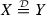:

Equality in distribution; X and Y have the same distribution.

$$X_{(i)}$$ :

ith order statistic of a set of random variables $$X_1,\ldots ,X_n$$, i.e., the ith smallest element of $$X_1,\ldots ,X_n$$.

$${\mathbbm {1}}_{\{E\}}$$ :

Indicator variable for event E, i.e., $${\mathbbm {1}}_{\{E\}}$$ is 1 if E occurs and 0 otherwise.

$$a^{\underline{b}}$$, $$a^{\overline{b}}$$ :

Factorial powers notation of Graham et al. ; “a to the b falling resp. rising”.

### Input to the Algorithm

n :

Length of the input array, i.e., the input size.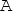:

Input array containing the itemsto be sorted; initially,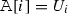.

$$U_i$$ :

ith element of the input, i.e., initially. We assume $$U_1,\ldots ,U_n$$ are i.i.d. $${\mathcal {U}}(0,1)$$ distributed.

### Notation Specific to the Algorithm

$$\varvec{\mathbf {t}} \in {\mathbb {N}}^3$$ :

Pivot sampling parameter, see Sect. 3.1.

$$k=k(\varvec{\mathbf {t}})$$ :

Sample size; defined in terms of $$\varvec{\mathbf {t}}$$ as $$k(\varvec{\mathbf {t}}) = t_1+t_2+t_3+2$$.

$$w$$ :

Insertionsort threshold; for $$n\le w$$, Quicksort recursion is truncated and we sort the subarray by Insertionsort.

M :

Cache size; the number of array elements that fit into the idealized cache; we assume $$M\ge B$$, $$B\mid M$$ (M is a multiple of B) and $$B\mid n$$; see Sect. 7.2.

B :

Block size; the number of array elements that fit into one cache block/line; see also M.

$$\mathrm {YQS}$$,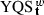:

Abbreviation for dual-pivot Quicksort with Yaroslavskiy’s partitioning method, where pivots are chosen by generalized pivot sampling with parameter $$\varvec{\mathbf {t}}$$ and where we switch to Insertionsort for subproblems of size at most $$w$$.

$$\mathrm {CQS}$$ :

Abbreviation for classic (single-pivot) Quicksort using Hoare’s partitioning, see e.g. [25, p. 329]; a variety of notations are with $$\mathrm {CQS}$$ in the superscript to denote the corresponding quantities for classic Quicksort, e.g.,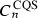is the number of (partitioning) comparisons needed by CQS on a random permutation of size n.

$$\varvec{\mathbf {V}} \in {\mathbb {N}}^k$$ :

(Random) sample for choosing pivots in the first partitioning step.

P, Q :

(Random) Values of chosen pivots in the first partitioning step.

Small element:

Element U is small if $$U<P$$.

Medium element:

Element U is medium if $$P<U<Q$$.

Large element:

Element U is large if $$Q < U$$.

Sampled-out element:

the $$k-2$$ elements of the sample that are not chosen as pivots.

Ordinary element:

The $$n-k$$ elements that have not been part of the sample.

k, g, $$\ell$$ :

Index variables used in Yaroslavskiy’s partitioning method, see Algorithm 1.

$$\mathcal {K}$$, $$\mathcal {G}$$, $$\mathcal {L}$$ :

Set of all (index) values attained by pointers k, g resp. $$\ell$$ during the first partitioning step; see Sect. 3.2 and proof of Lemma 5.1.

$$c\text{@ }\mathcal {P}$$ :

$$c\in \{s,m,l\}$$, $$\mathcal {P} \subset \{1,\ldots ,n\}$$ (random) number of c-type (small, medium or large) elements that are initially located at positions in $$\mathcal {P}$$, i.e., $$c\text{@ }\mathcal {P} \,{=}\, \bigl |\{ i \in \mathcal {P} : U_i \text { has type } c \}\bigr |.$$

$$l\text{@ }\mathcal {K}$$, $$s\text{@ }\mathcal {K}$$, $$s\text{@ }\mathcal {G}$$ :

See $$c\text{@ }\mathcal {P}$$

$$\chi$$ :

(Random) point where k and g first meet.

$$\delta$$ :

Indicator variable of the random event that $$\chi$$ is on a large element, i.e., $$\delta = {\mathbbm {1}}_{\{U_\chi > Q\}}$$.

$$C_n^{{\mathtt {type}}}$$ :

With $${\mathtt {type}}\in \{{\mathtt {root}},{\mathtt {left}},{\mathtt {middle}},{\mathtt {right}}\}$$; (random) costs of a (recursive) call to GeneralizedYaroslavskiy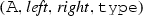where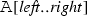contains n elements, i.e., $$right - left +1 = n$$. The array elements are assumed to be in random order, except for the $$t_1$$, resp. $$t_2$$ leftmost elements for $$C_n^{{\mathtt {left}}}$$ and $$C_n^{{\mathtt {middle}}}$$ and the $$t_3$$ rightmost elements for $$C_n^{{\mathtt {right}}}$$; for all $$\mathtt {type}$$s holds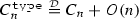, see Sect. 5.1.

$$T_n^{{\mathtt {type}}}$$ :

With $${\mathtt {type}}\in \{{\mathtt {root}},{\mathtt {left}},{\mathtt {middle}},{\mathtt {right}}\}$$; the costs of the first partitioning step of a call to GeneralizedYaroslavskiy; for all $$\mathtt {type}$$s holds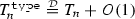, see Sect. 5.1.

$$T_n$$ :

The costs of the first partitioning step, where only costs of procedure Partition are counted, see Sect. 5.1.

$$W_n^{{\mathtt {type}}}$$ :

With $${\mathtt {type}}\in \{{\mathtt {root}},{\mathtt {left}},{\mathtt {middle}},{\mathtt {right}}\}$$; as $$C_n^{{\mathtt {type}}}$$, but the calls are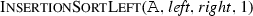for $$W_n^{{\mathtt {root}}}$$,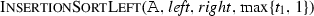for $$W_n^{{\mathtt {left}}}$$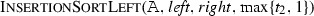for $$W_n^{{\mathtt {middle}}}$$ and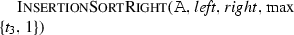for $$W_n^{{\mathtt {right}}}$$.

$$W_n$$ :

(Random) Costs of sorting a random permutation of size n with Insertionsort.

$$C_n$$, $$S_n$$, $${ BC }_n$$, $${ SE }_n$$ :

(Random) Number of comparisons / swaps / Bytecodes / scanned elements ofon a random permutation of size n that are caused in procedure Partition; see Sect. 1.1 for more information on the cost measures; in Sect. 5.1, $$C_n$$ is used as general placeholder for any of the above cost measures.

$$T_{C}$$, $$T_{S}$$, $$T_{{ BC }}$$, $$T_{{ SE }}$$ :

(Random) Number of comparisons / swaps / Bytecodes / element scans of the first partitioning step ofon a random permutation of size n; $$T_{C}(n)$$, $$T_{S}(n)$$ and $$T_{{ BC }}(n)$$ when we want to emphasize dependence on n.

$$a_C$$, $$a_S$$, $$a_{{ BC }}$$, $$a_{{ SE }}$$ :

Coefficient of the linear term of $$\mathop {{\mathbb {E}}}\nolimits [T_{C}(n)]$$, $$\mathop {{\mathbb {E}}}\nolimits [T_{S}(n)]$$, $$\mathop {{\mathbb {E}}}\nolimits [T_{{ BC }}(n)]$$ and $$\mathop {{\mathbb {E}}}\nolimits [T_{{ SE }}(n)]$$; see Theorem 4.1.

$${\mathcal {H}}$$ :

Discrete entropy; defined in Eq. (1).

$${\mathcal {H}}^{*}(\varvec{\mathbf {p}})$$ :

Continuous (Shannon) entropy with basis e; defined in Eq. (2).

$$\varvec{\mathbf {J}}\in {\mathbb {N}}^3$$ :

(Random) Vector of subproblem sizes for recursive calls; for initial size n, we have $$\varvec{\mathbf {J}} \in \{0,\ldots ,n-2\}^3$$ with $$J_1+J_2+J_3 = n-2$$.

$$\varvec{\mathbf {I}}\in {\mathbb {N}}^3$$ :

(Random) Vector of partition sizes, i.e., the number of small, medium resp. large ordinary elements; for initial size n, we have $$\varvec{\mathbf {I}} \in \{0,\ldots ,n-k\}^3$$ with $$I_1+I_2+I_3 = n-k$$; $$\varvec{\mathbf {J}} = \varvec{\mathbf {I}} + \varvec{\mathbf {t}}$$ and conditional on $$\varvec{\mathbf {D}}$$ we have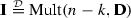.

$$\varvec{\mathbf {D}}\in {[}0,1{]}^3$$ :

(Random) Spacings of the unit interval (0, 1) induced by the pivots P and Q, i.e., $$\varvec{\mathbf {D}} = (P,Q-P,1-Q)$$;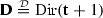.

$$a^*_C$$, $$a^*_S$$, $$a^*_{{ BC }}$$, $$a^*_{ SE }$$ :

Limit of $$a_C$$, $$a_S$$, $$a_{{ BC }}$$ resp. $$a_{{ SE }}$$ for the optimal sampling parameter $$\varvec{\mathbf {t}}$$ when $$k\rightarrow \infty$$.

$$\varvec{\mathbf {\tau }}_C^*,\, \varvec{\mathbf {\tau }}_S^*,\, \varvec{\mathbf {\tau }}_{{ BC }}^*,\, \varvec{\mathbf {\tau }}_{{ SE }}^*$$ :

Optimal limiting ratio $$\varvec{\mathbf {t}} / k \rightarrow \varvec{\mathbf {\tau }}_C^*$$ such that $$a_C \rightarrow a^*_C$$ (resp. for S, $${ BC }$$ and $${ SE }$$).

### Appendix 2: Properties of Distributions

We herein collect definitions and basic properties of the distributions used in this paper. They will be needed for computing expected values in Appendix 3. This appendix is an update of Appendix C in , which we include here for the reader’s convenience.

We use the notation $$x^{\overline{n}}$$ and $$x^{\underline{n}}$$ of Graham et al.  for rising and falling factorial powers, respectively.

### Dirichlet Distribution and Beta Function

For $$d\in {\mathbb {N}}$$ let $$\Delta _d$$ be the standard $$(d-1)$$-dimensional simplex, i.e.,

\begin{aligned} \Delta _d&\,{:=}\,\biggl \{ x = (x_1,\ldots ,x_d) \,{:}\, \forall i : x_i \ge 0 \; \,{\wedge }\,\sum _{1\le i \le d} x_i = 1 \biggr \}. \end{aligned}
(10)

Let $$\alpha _1,\ldots ,\alpha _d > 0$$ be positive reals. A random variable $$\varvec{\mathbf {X}} \in {\mathbb {R}}^d$$ is said to have the Dirichlet distribution with shape parameter $$\varvec{\mathbf {\alpha }}:=(\alpha _1,\ldots ,\alpha _d)$$—abbreviated as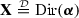—if it has a density given by

\begin{aligned} f_{\varvec{\mathbf {X}}}(x_1,\ldots ,x_d)&\,{:=}\,{\left\{ \begin{array}{ll} \frac{1}{\mathrm {B}(\varvec{\mathbf {\alpha }})} \cdot x_1^{\alpha _1 - 1} \cdots x_d^{\alpha _d-1} , &{} \text {if } \varvec{\mathbf {x}} \in \Delta _d ; \\ 0 , &{} \text {otherwise} . \end{array}\right. } \end{aligned}
(11)

Here, $$\mathrm {B}(\varvec{\mathbf {\alpha }})$$ is the d-dimensional Beta function defined as the following Lebesgue integral:

\begin{aligned} \mathrm {B}(\alpha _1,\ldots ,\alpha _d)&\,{:=}\,\int _{\Delta _d} x_1^{\alpha _1 - 1} \cdots x_d^{\alpha _d-1} \; \mu (d \varvec{\mathbf {x}}). \end{aligned}
(12)

The integrand is exactly the density without the normalization constant $$\frac{1}{\mathrm {B}(\alpha )}$$, hence $$\int f_X \,d\mu = 1$$ as needed for probability distributions.

The Beta function can be written in terms of the Gamma function $$\Gamma (t) = \int _0^\infty x^{t-1} e^{-x} \,dx$$ as

\begin{aligned} \mathrm {B}(\alpha _1,\ldots ,\alpha _d)&\,{=}\, \frac{\Gamma (\alpha _1) \cdots \Gamma (\alpha _d)}{\Gamma (\alpha _1+\cdots +\alpha _d)}. \end{aligned}
(13)

(For integral parameters $$\varvec{\mathbf {\alpha }}$$, a simple inductive argument and partial integration suffice to prove (13).)

Note that $${\mathrm {Dir}}(1,\ldots ,1)$$ corresponds to the uniform distribution over $$\Delta _d$$. For integral parameters $$\varvec{\mathbf {\alpha }}\in {\mathbb {N}}^d$$, $${\mathrm {Dir}}(\varvec{\mathbf {\alpha }})$$ is the distribution of the spacings or consecutive differences induced by appropriate order statistics of i.i.d. uniformly in (0, 1) distributed random variables, as summarized in the following proposition.

### Proposition 9.1

(, Section 6.4) Let $$\varvec{\mathbf {\alpha }}\in {\mathbb {N}}^d$$ be a vector of positive integers and set $$k :=-1 + \sum _{i=1}^d \alpha _i$$. Further let $$V_1,\ldots ,V_{k}$$ be k random variables i.i.d. uniformly in (0, 1) distributed. Denote by $$V_{(1)}\le \cdots \le V_{(k)}$$ their corresponding order statistics. We select some of the order statistics according to $$\varvec{\mathbf {\alpha }}$$: for $$j=1,\ldots ,d-1$$ define $$W_j :=V_{(p_j)}$$, where $$p_j :=\sum _{i=1}^j \alpha _i$$. Additionally, we set $$W_0 :=0$$ and $$W_d :=1$$.

Then, the consecutive distances (or spacings) $$D_j :=W_j - W_{j-1}$$ for $$j=1,\ldots ,d$$ induced by the selected order statistics $$W_1,\ldots ,W_{d-1}$$ are Dirichlet distributed with parameter $$\varvec{\mathbf {\alpha }}$$: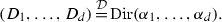In the computations of Sect. 6.1, mixed moments of Dirichlet distributed variables will show up, which can be dealt with using the following general statement.

### Lemma 9.2

Let $$\varvec{\mathbf {X}} = (X_1,\ldots ,X_d) \in {\mathbb {R}}^d$$ be a $${\mathrm {Dir}}(\varvec{\mathbf {\alpha }})$$ distributed random variable with parameter $$\varvec{\mathbf {\alpha }}= (\alpha _1,\ldots ,\alpha _d)$$. Let further $$m_1,\ldots ,m_d \in {\mathbb {N}}$$ be non-negative integers and abbreviate the sums $$A :=\sum _{i=1}^d \alpha _i$$ and $$M :=\sum _{i=1}^d m_i$$. Then we have

\begin{aligned} \mathop {{\mathbb {E}}}\nolimits \bigl [ X_1^{m_1} \cdots X_d^{m_d} \bigr ]&\,{=}\, \frac{\alpha _1^{\overline{m_1}} \cdots \alpha _d^{\overline{m_d}}}{A^{\overline{M}}}. \end{aligned}

### Proof

Using $$\frac{\Gamma (z+n)}{\Gamma (z)} = z^{\overline{n}}$$ for all $$z\in {\mathbb {R}}_{>0}$$ and $$n \in {\mathbb {N}}$$, we compute

\begin{aligned} \mathop {{\mathbb {E}}}\nolimits \bigl [ X_1^{m_1} \cdots X_d^{m_d} \bigr ]&\,{=}\, \int _{\Delta _d} x_1^{m_1} \cdots x_d^{m_d} \cdot \frac{x_1^{\alpha _1-1} \cdots x_d^{\alpha _d-1}}{\mathrm {B}(\varvec{\mathbf {\alpha }})} \; \mu (dx) \end{aligned}
(14)
\begin{aligned}&\,{=}\, \frac{\mathrm {B}(\alpha _1 + m_1,\ldots ,\alpha _d + m_d)}{\mathrm {B}(\alpha _1,\ldots ,\alpha _d)}\end{aligned}
(15)
\begin{aligned}&\,{(13)}\, \frac{ \alpha _1^{\overline{m_1}} \cdots \alpha _d^{\overline{m_d}} }{ A^{\overline{M}} }. \end{aligned}
(16)

$$\square$$

For completeness, we state here a two-dimensional Beta integral with an additional logarithmic factor that is needed in Appendix 4 (see also , Appendix B):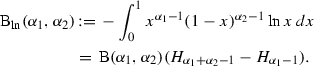(17)

For integral parameters $$\varvec{\mathbf {\alpha }}$$, the proof is elementary: By partial integration, we can find a recurrence equation for $$\mathrm {B}_{\ln }$$:

\begin{aligned} \mathrm {B}_{\ln }(\alpha _1,\alpha _2)&\,{=}\, \frac{1}{\alpha _1} \mathrm {B}(\alpha _1,\alpha _2) \,{+}\, \frac{\alpha _2-1}{\alpha _1} \mathrm {B}_{\ln }(\alpha _1+1,\alpha _2-1). \end{aligned}

Iterating this recurrence until we reach the base case $$\mathrm {B}_{\ln }(a,0) = \frac{1}{a^2}$$ and using (13) to expand the Beta function, we obtain (17).

### Multinomial Distribution

Let $$n,d \in {\mathbb {N}}$$ and $$k_1,\ldots ,k_d\in {\mathbb {N}}$$. Multinomial coefficients are the multidimensional extension of binomials:

\begin{aligned} \left( {\begin{array}{c}n\\ k_1,k_2,\ldots ,k_d\end{array}}\right)&\,{:=}\,{\left\{ \begin{array}{ll} \displaystyle \frac{n!}{k_1! k_2! \cdots k_d!} , &{} \displaystyle \text {if } n=\sum _{i=1}^d k_i ; \\ 0 , &{} \text {otherwise} . \end{array}\right. } \end{aligned}

Combinatorially, $$\left( {\begin{array}{c}n\\ k_1,\ldots ,k_d\end{array}}\right)$$ is the number of ways to partition a set of n objects into d subsets of respective sizes $$k_1,\ldots ,k_d$$ and thus they appear naturally in the multinomial theorem:

\begin{aligned} (x_1 + \cdots + x_d)^n&\,{=}\, \sum _{\begin{array}{c} i_1,\ldots ,i_d \in {\mathbb {N}}\\ i_1+\cdots +i_d = n \end{array}} \left( {\begin{array}{c}n\\ i_1,\ldots ,i_d\end{array}}\right) \; x_1^{i_1} \cdots x_d^{i_d} \quad \text {for } n\in {\mathbb {N}}. \end{aligned}
(18)

Let $$p_1,\ldots ,p_d \in [0,1]$$ such that $$\sum _{i=1}^d p_i = 1$$. A random variable $$\varvec{\mathbf {X}}\in {\mathbb {N}}^d$$ is said to have multinomial distribution with parameters n and $$\varvec{\mathbf {p}} = (p_1,\ldots ,p_d)$$—written shortly as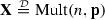—if for any $$\varvec{\mathbf {i}} = (i_1,\ldots ,i_d) \in {\mathbb {N}}^d$$ holds

\begin{aligned} {\mathbb {P}}(\varvec{\mathbf {X}} = \varvec{\mathbf {i}})&\,{=}\, \left( {\begin{array}{c}n\\ i_1,\ldots ,i_d\end{array}}\right) \; p_1^{i_1} \cdots p_d^{i_d}. \end{aligned}

We need some expected values involving multinomial variables. They can be expressed as special cases of the following mixed factorial moments.

### Lemma 9.3

Let $$p_1,\ldots ,p_d \in [0,1]$$ such that $$\sum _{i=1}^d p_i =1$$ and consider a $${\mathrm {Mult}}(n,\varvec{\mathbf {p}})$$ distributed variable $$\varvec{\mathbf {X}} = (X_1,\ldots ,X_d) \in {\mathbb {N}}^d$$. Let further $$m_1,\ldots ,m_d \in {\mathbb {N}}$$ be non-negative integers and abbreviate their sum as $$M :=\sum _{i=1}^d m_i$$. Then we have

\begin{aligned} \mathop {{\mathbb {E}}}\nolimits \bigl [ (X_1)^{\underline{m_1}} \cdots (X_d)^{\underline{m_d}} \bigr ]&\,{=}\, n^{\underline{M}} \, p_1^{m_1} \cdots p_d^{m_d}. \end{aligned}

### Proof

We compute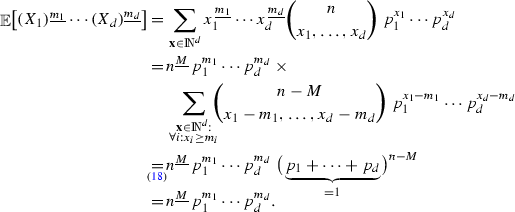(19)

$$\square$$

### Appendix 3: Proof of Lemma 6.1

In this appendix, we give the computations needed to prove Lemma 6.1. They were also given in Appendix D of , but we reproduce them here for the reader’s convenience.

We recall thatandand start with the simple ingredients: $$\mathop {{\mathbb {E}}}\nolimits [I_j]$$ for $$j=1,2,3$$.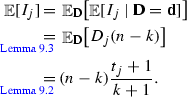(20)

The term $$\mathop {{\mathbb {E}}}\nolimits \bigl [{\mathrm {B}}\bigl (\frac{I_3}{n-k}\bigr )\bigr ]$$ is then easily computed using (20):

\begin{aligned} \mathop {{\mathbb {E}}}\nolimits \bigl [{\mathrm {B}}\bigl (\tfrac{I_3}{n-k}\bigr )\bigr ]&\,{=}\, \frac{\mathop {{\mathbb {E}}}\nolimits [{I_3}]}{n-k} \,{=}\, \frac{t_3+1}{k+1} \,{\,{=}\,}\, \Theta (1). \end{aligned}
(21)

This leaves us with the hypergeometric variables; using the well-known formula $$\mathop {{\mathbb {E}}}\nolimits [{\mathrm {HypG}}(k,r,n)] = k\frac{r}{n}$$, we find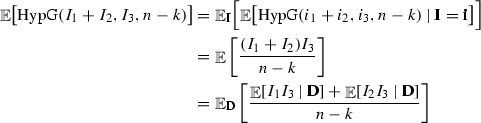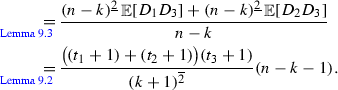(22)

The second hypergeometric summand is obtained similarly. $$\square$$

### Appendix 4: Solution to the Recurrence

This appendix is an update of Appendix E in , we include it here for the reader’s convenience.

An elementary proof can be given for Theorem 6.2 using Roura ’s Continuous Master Theorem (CMT) . The CMT applies to a wide class of full-history recurrences whose coefficients can be well-approximated asymptotically by a so-called shape function $$w:[0,1] \rightarrow {\mathbb {R}}$$. The shape function describes the coefficients only depending on the ratio j / n of the subproblem size j and the current size n (not depending on n or j itself) and it smoothly continues their behavior to any real number $$z\in [0,1]$$. This continuous point of view also allows to compute precise asymptotics for complex discrete recurrences via fairly simple integrals.

### Theorem 9.4

(, Theorem 18) Let $$F_n$$ be recursively defined by

\begin{aligned} F_n \,{=}\, {\left\{ \begin{array}{ll} b_n, &{}\text {for }~ 0 \le n < N; \\ \displaystyle {t_n \,{+}\, {\sum _{j=0}^{n-1} w_{n,j} \, F_j}, } &{}\text {for }~ n \ge N\, \end{array}\right. } \end{aligned}
(23)

where the toll function satisfies $$t_n \sim K n^\alpha \log ^\beta (n)$$ as $$n\rightarrow \infty$$ for constants $$K\ne 0$$, $$\alpha \ge 0$$ and $$\beta > -1$$. Assume there exists a function $$w:[0,1]\rightarrow {\mathbb {R}}$$, such that

\begin{aligned} \sum _{j=0}^{n-1} \,\biggl | w_{n,j} \,{-}\, \int _{j/n}^{(j+1)/n} w(z) \, dz \biggr | \,{=}\, O(n^{-d}), \qquad \qquad (n\rightarrow \infty ), \end{aligned}
(24)

for a constant $$d>0$$. With $$H :=1 - \int _0^1 z^\alpha w(z) \, dz$$, we have the following cases:

1. 1.

If $$H > 0$$, then $$F_n \sim \frac{t_n}{H}$$.

2. 2.

If $$H = 0$$, then $$F_n \sim \frac{t_n \ln n}{\tilde{H}}$$ with $$\tilde{H} = -(\beta +1)\int _0^1 z^\alpha \ln (z) \, w(z) \, dz$$.

3. 3.

If $$H < 0$$, then $$F_n \sim \Theta (n^c)$$ for the unique $$c\in {\mathbb {R}}$$ with $$\int _0^1 z^c w(z) \, dz = 1$$.

The analysis of single-pivot Quicksort with pivot sampling is the application par excellence for the CMT . We will generalize this work of Martínez and Roura to the dual-pivot case.

Note that the recurrence for $$F_n$$ depends linearly on $$t_n$$, so whenever $$t_n = t'_n + t_n^{\prime \prime }$$, we can apply the CMT to both the summands of the toll function separately and sum up the results. In particular, if we have an asymptotic expansion for $$t_n$$, we get an asymptotic expansion for $$F_n$$; the latter might however get truncated in precision when we end up in case 3 of Theorem 9.4.

Our Eq. (6) has the form of (23) with

\begin{aligned} w_{n,j} \,{=}\, \sum _{r=1}^3 {\mathbb {P}}\bigl ( J_r = j \bigr ). \end{aligned}

Recall that $$\varvec{\mathbf {J}} = \varvec{\mathbf {I}} + \varvec{\mathbf {t}}$$ and thatconditional on $$\varvec{\mathbf {D}}$$, which in turn is a random variable with distribution.

The probabilities $${\mathbb {P}}(J_r = j) = {\mathbb {P}}(I_r = j-t_r)$$ can be computed using that the marginal distribution of $$I_r$$ is binomial $${\mathrm {Bin}}(N,D_r)$$, where we abbreviate by $$N :=n-k$$ the number of ordinary elements. It is convenient to consider $${\tilde{\mathbf{D}}} :=(D_r,1-D_r)$$, which is distributed like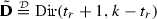. For $$i\in [0..N]$$ holds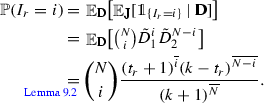(25)

### Finding a Shape Function

In general, a good guess for the shape function is $$w(z) = \lim _{n\rightarrow \infty } n\,w_{n,zn}$$  and, indeed, this will work out for our weights. We start by considering the behavior for large n of the terms $${\mathbb {P}}(I_r = zn + \rho )$$ for $$r=1,2,3$$, where $$\rho$$ does not depend on n. Assuming $$zn+\rho \in \{0,\ldots ,n\}$$, we compute

\begin{aligned}&{\mathbb {P}}(I_r=zn+\rho ) \,{=}\, \left( {\begin{array}{c}N\\ zn+\rho \end{array}}\right) \frac{(t_r+1)^{\overline{zn+\rho }}(k-t_r)^{\overline{(1-z)n-\rho }}}{(k+1)^{\overline{N}}} \nonumber \\&\,{=}\, \frac{N!}{(zn+\rho )!((1-z)n-\rho )!} \frac\frac{(zn + \rho + t_r)!}{t_r!} \, \frac{\bigl ((1-z)n - \rho + k - t_r - 1\bigr )!}{(k-t_r-1)!}}\frac{(k+N)!}{k!}} \nonumber \\&\,{=}\, \underbrace{\frac{k!}{t_r!(k-t_r-1)!}} _ {{}= 1/\mathrm {B}(t_r+1,k-t_r)} \frac{(zn+\rho +t_r)^{\underline{t_r}} \, \bigl ((1-z)n - \rho + k - t_r - 1\bigr )^{\underline{k-t_r-1}}}{n^{\underline{k}}}, \end{aligned
(26)

and since this is a rational function in n,

\begin{aligned}&\,{=}\, \frac{1}{\mathrm {B}(t_r+1,k-t_r)} \frac{ (zn)^{t_r} ((1-z)n)^{k-t_r-1}}{n^k} \cdot \Bigl ( 1 \,{+}\, O(n^{-1}) \Bigr ) \nonumber \\&\,{=}\, \underbrace{ \frac{1}{\mathrm {B}(t_r+1,k-t_r)} z^{t_r} (1-z)^{k-t_r-1} } _ {=:w_r(z)} \cdot \Bigl ( n^{-1} \,{+}\, O(n^{-2}) \Bigr ), \qquad (n\rightarrow \infty ). \end{aligned}
(27)

Thus $$n{\mathbb {P}}(J_r = zn) \,{=}\, n{\mathbb {P}}(I_r = zn-t_r) \,{\sim }\,w_r(z)$$, and our candidate for the shape function is

\begin{aligned} w(z) \,{=}\, \sum _{r=1}^3 w_r(z) \,{=}\, \sum _{r=1}^3 \frac{z^{t_r}(1-z)^{k-t_r-1}}{\mathrm {B}(t_r+1,k-t_r)}. \end{aligned}

Note that $$w_r(z)$$ is the density function of a $${\mathrm {Dir}}(t_r+1,k-t_r)$$ distributed random variable.

It remains to verify condition (24). We first note using (27) that

\begin{aligned} n w_{n,zn} \,{=}\, w(z) \,{+}\, O(n^{-1}). \end{aligned}
(28)

Furthermore as w(z) is a polynomial in z, its derivative exists and is finite in the compact interval [0, 1], so its absolute value is bounded by a constant $$C_w$$. Thus $$w:[0,1]\rightarrow {\mathbb {R}}$$ is Lipschitz-continuous with Lipschitz constant $$C_w$$:

\begin{aligned} \forall z,z'\in [0,1]&\,{:}\, \bigl |w(z) - w(z')\bigr | \,{\le }\,C_w |z-z'|. \end{aligned}
(29)

For the integral from (24), we then have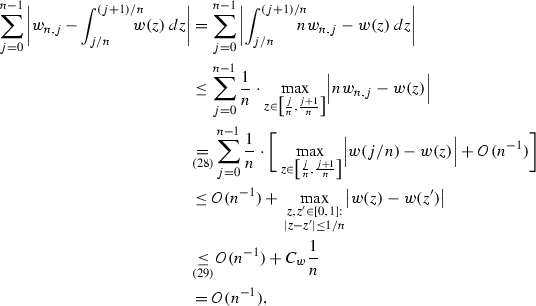which shows that our w(z) is indeed a shape function of our recurrence (with $$d=1$$).

### Applying the CMT

With the shape function w(z) we can apply Theorem 9.4 with $$\alpha =1$$, $$\beta =0$$ and $$K=a$$. It turns out that case 2 of the CMT applies:

\begin{aligned} H&\,{=}\, 1 \,{-}\, \int _0^1 z \, w(z) \, dz\\&\,{=}\, 1 \,{-}\,\sum _{r=1}^3 \int _0^1 z \, w_r(z) \, dz\\&\,{=}\, 1 \,{-}\,\sum _{r=1}^3 \frac{1}{\mathrm {B}(t_r+1,k-t_r)} \mathrm {B}(t_r+2,k-t_r)\\&{\mathop {=}\limits _{(13)}} 1 \,{-}\, \sum _{r=1}^3\frac{t_r+1}{k+1} \,{=}\, 0. \end{aligned}

For this case, the leading-term coefficient of the solution is $$t_n \ln (n) / \tilde{H} = n \ln (n) / \tilde{H}$$ with

\begin{aligned} \tilde{H}&\,{=}\, - \int _0^1 z \ln (z) \, w(z) \, dz\\&\,{=}\, \sum _{r=1}^3 \frac{1}{\mathrm {B}(t_r+1,k-t_r)} \mathrm {B}_{\ln }(t_r+2,k-t_r)\\&{\mathop {=}\limits _{(17)}} \sum _{r=1}^3 \frac{\mathrm {B}(t_r+2,k-t_r)(H_{k+1} - H_{t_r+1})}{\mathrm {B}(t_r+1,k-t_r)}\\&\,{=}\, \sum _{r=1}^3 \frac{t_r+1}{k+1}(H_{k+1} - H_{t_r+1}). \end{aligned}

So indeed, we find $$\tilde{H} = {\mathcal {H}}$$ as claimed in Theorem 6.2, concluding the proof for the leading term.

As argued above, the error bound is obtained by a second application of the CMT, where the toll function now is $$K\cdot n^{1-\epsilon }$$ for a K that gives an upper bound of the toll function: $$\mathop {{\mathbb {E}}}\nolimits [T_n] - an \le K n^{1-\epsilon }$$ for large n. We thus apply Theorem 9.4 with $$\alpha =1-\epsilon$$, $$\beta =0$$ and K. We note that $$f_c : {\mathbb {R}}_{\ge 1} \rightarrow {\mathbb {R}}$$ with $$f_c(z) = \Gamma (z)/\Gamma (z+c)$$ is a strictly decreasing function in z for any positive fixed c and hence the beta function $$\mathrm {B}$$ is strictly decreasing in all its arguments by (13). With that, we compute

\begin{aligned} H&\,{=}\, 1 \,{-}\, \int _0^1 z^{1-\epsilon } \, w(z) \, dz\\&\,{=}\, 1 \,{-}\,\sum _{r=1}^3 \frac{\mathrm {B}(t_r+2-\epsilon ,k-t_r)}{\mathrm {B}(t_r+1,k-t_r)}\\&\,{<}\, 1 \,{-}\,\sum _{r=1}^3 \frac{\mathrm {B}(t_r+2,k-t_r)}{\mathrm {B}(t_r+1,k-t_r)} \,{=}\,0. \end{aligned}

Consequently, case 3 applies. We already know from above that the exponent that makes H become 0 is $$\alpha =1$$, so the $$F_n = \Theta (n)$$. This means that a toll function that is bounded by $$O(n^{1-\epsilon })$$ for $$\epsilon >0$$ contributes only to the linear term in overall costs of Quicksort, and this is independent of the pivot sampling parameter $$\varvec{\mathbf {t}}$$. Putting both results together yields Theorem 6.2.

Note that the above arguments actually derive—not only prove correctness of—the precise leading-term asymptotics of a quite involved recurrence equation. Compared with Hennequin’s original proof via generating functions, it needed less mathematical theory.

## Rights and permissions

Reprints and Permissions